## Volume 15 - Issue 3

Research Article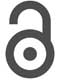Creative Commons, CC-BY

# Nano Drive for Biomedical Science and Research

*Corresponding author: Afonin Sergey Mikhailovich, National Research University of Electronic Technology, MIET, 124498, Moscow, Russia.

Received: November 30, 2021; Published: January 19, 2022

#### Abstract

The structural model of the nano drive is obtained. The structural scheme of the nano drive is constructed. In biomedical science and research for the nano systems with the nano drive its deformations are determined.

Keywords: Nano drive; Nano piezo drive; Deformation; Matrix equation; Structural model; Biomedical science and research

#### Introduction

Nano piezo drive on the reverse piezo effect is applied for conversion energy in the nano systems for biomedical science and research [1-8]. on the piezoelectric or electrostriction effects are used for nano movements in the precision equipment. The energy conversion in the structural scheme of the nano drive is visibility and logical in the difference from the conversion from the Cady’s and Mason’s schemes [7-9]. The structural model and scheme of nano drive are constructed from it matrix equations and differential equation for the drive [8-16].

Nano drives are used for atomic force microscopy, nano manipulators, nanotechnology, biotechnology, astronomy, space research, metrology, laser resonator [16-25].

#### Model of Nano Drive

Let us consider the deformation of the nano drives for biomedical science and research. Two matrix equations [8,11-16] for the nano piezo drive have the form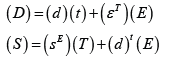Where (D), (S), (T), (E) are matrices for electric induction, relative deformation, mechanical field and electric field stresses, t is matrix transpose operator.

Matrices for the piezo modules, the dielectric constants and the elastic compliances for the nano piezo drive from PZT have the form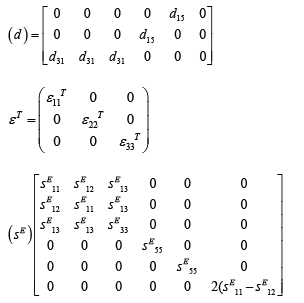The equation of the reverse piezo effect [8, 11-16] for nano piezo drive on Figure 1 has the formwhere m, i, j are axises (Figure 1).

Differential equation for nano drive in nano biomedical science and research has the form [11-48].where x is coordinate, is operator, are coefficients, is speed of sound.

The equations structural model for nano drive has the formis the control parameter on Figure 2, is the length nano drive, are the masses.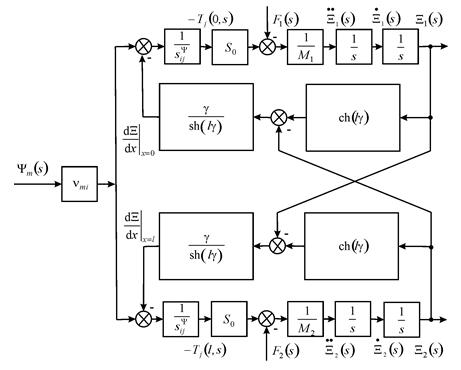Figure 2: Structural scheme nano drive for nano biomedical science and research.

Structural scheme nano drive on Figure 2 is used for calculation its deformations in nano systems for biomedical science and research instead Cady’s and Mason’s schemes (Figure 2).

#### Deformations of Nano Drive

Therefore, for nano drive its matrix equation deformations has the form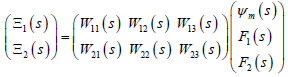The steady state for its deformations have the formThe steady state for its deformations for the transverse piezo drive have the form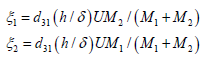For the nano piezo drive from PZT = 0.25 nm/V, =10, = 0.5 kg, = 2 kg and = 50 V its steady-state deformations are determined in the form = 100 nm, = 25 nm, = 125 nm. Theoretical and practical deformations for nano piezo drive are coincidences with an error of 10%.

#### Conclusions

The structural model for nano drive is determined from matrix equations and differential equation for the drive. The equations structural model for nano drive are obtained for nano systems in biomedical science and research.